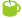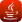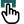### Found in titles:

1.Jython Example: canvas2D_polar1_waterloo.py [100%] Plotting X-Y in polar coordinates
2.Jython Example: func_nonparametric1.py [100%] Defining and plotting a non-parametric function
3.Jython Example: real_life_AA518L8Fig3.py [83%] Plotting H on PlotXY for paper AA518L8Fig3
4.Jython Example: data_p1d_1.py [83%] Data in X-Y, plotting on 2D canvas
5.Jython Example: io_read_csvdata.py [83%] Reading a CSV file and plotting columns
6.Java Example: jaida_PlotWithStyles.java [83%] Plotting in Jaida with different styles
7.Jython Example: canvas2D_polar2_waterloo.py [83%] Plotting X-Y using polar coordinates
8.Jython Example: canvas2D_points_waterloo.py [83%] Plotting X-Y using Waterloo scientific library
9.Jython Example: jaida_Tuple.py [83%] Plotting histograms and data arrays using Jaida
10.Jython Example: data_p1d_2.py [66%] Data in X-Y with error on Y, plotting on 2D canvas
11.Java Example: jaida_Tuple.java [66%] Plotting histograms and data arrays using Jaida in Java
12.Wiki: DMelt:JMathlab/Plotting [100%] (in title)
Searching 62556 articles took 9 ms